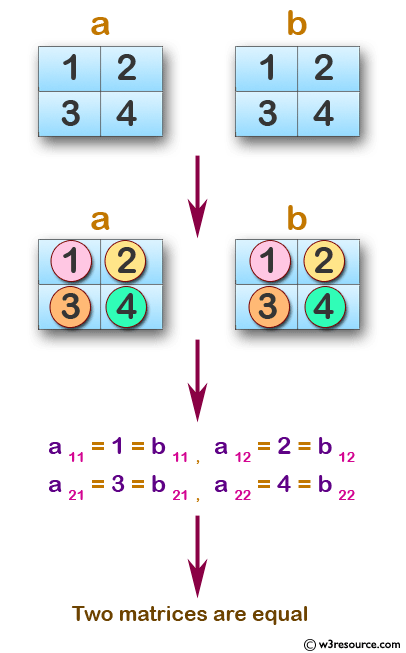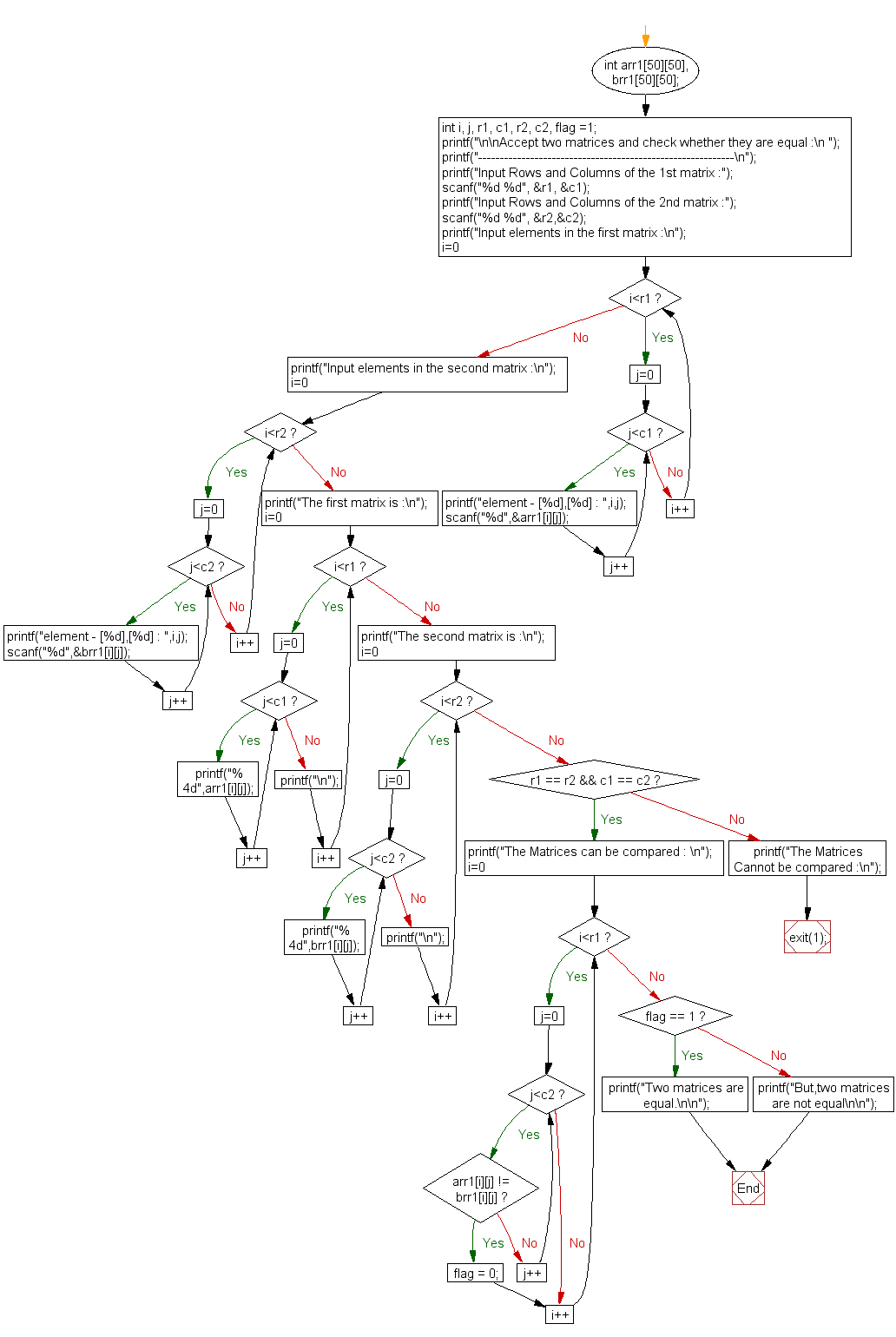﻿ C exercises: Accept two matrices and check whether they are equal - w3resource# C Exercises: Accept two matrices and check whether they are equal

## C Array: Exercise-30 with Solution

Write a program in C to accept two matrices and check whether they are equal.

Pictorial Presentation:Sample Solution:

C Code:

``````#include <stdio.h>
#include <stdlib.h>

void main()
{
int arr1, brr1;
int i, j, r1, c1, r2, c2, flag =1;

printf("\n\nAccept two matrices and check whether they are equal :\n ");
printf("-----------------------------------------------------------\n");

printf("Input Rows and Columns of the 1st matrix :");
scanf("%d %d", &r1, &c1);

printf("Input Rows and Columns of the 2nd matrix :");
scanf("%d %d", &r2,&c2);
printf("Input elements in the first matrix :\n");
for(i=0;i<r1;i++)
{
for(j=0;j<c1;j++)
{
printf("element - [%d],[%d] : ",i,j);
scanf("%d",&arr1[i][j]);
}
}
printf("Input elements in the second matrix :\n");
for(i=0;i<r2;i++)
{
for(j=0;j<c2;j++)
{
printf("element - [%d],[%d] : ",i,j);
scanf("%d",&brr1[i][j]);
}
}
printf("The first matrix is :\n");
for(i=0;i<r1;i++)
{
for(j=0;j<c1 ;j++)
printf("% 4d",arr1[i][j]);
printf("\n");
}
printf("The second matrix is :\n");
for(i=0;i<r2;i++)
{
for(j=0;j<c2 ;j++)
printf("% 4d",brr1[i][j]);
printf("\n");
}
/* Comparing two matrices for equality */

if(r1 == r2 && c1 == c2)
{
printf("The Matrices can be compared : \n");
for(i=0; i<r1; i++)
{
for(j=0; j<c2; j++)
{
if(arr1[i][j] != brr1[i][j])
{
flag = 0;
break;
}
}
}
}
else
{  printf("The Matrices Cannot be compared :\n");
exit(1);
}
if(flag == 1 )
printf("Two matrices are equal.\n\n");
else
printf("But,two matrices are not equal\n\n");
}
```
```

Sample Output:

```Accept two matrices and check whether they are equal :
-----------------------------------------------------------
Input Rows and Columns of the 1st matrix :2 2
Input Rows and Columns of the 2nd matrix :2 2
Input elements in the first matrix :
element - , : 1
element - , : 2
element - , : 3
element - , : 4
Input elements in the second matrix :
element - , : 1
element - , : 2
element - , : 3
element - , : 4
The first matrix is :
1   2
3   4
The second matrix is :
1   2
3   4
The Matrices can be compared :
Two matrices are equal.
```

Flowchart:C Programming Code Editor:

Improve this sample solution and post your code through Disqus.

What is the difficulty level of this exercise?

﻿